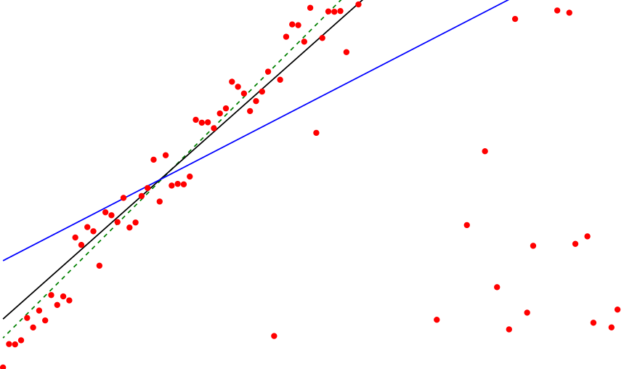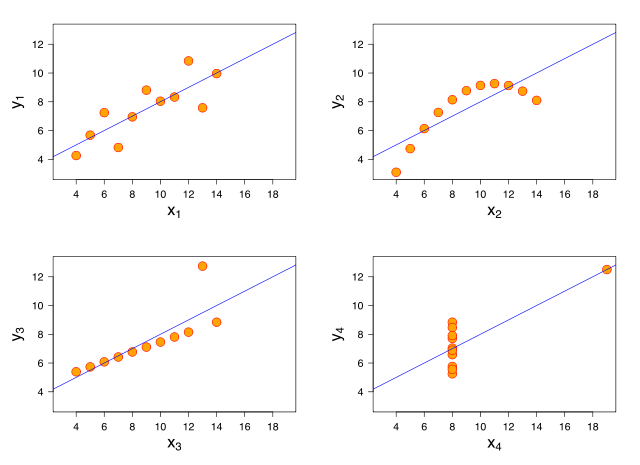Are you more of a visual learner? Check out our online video lectures and start your statistics course now for free!Image: “The Thiel–Sen estimator (black line) of a set of sample points, compared to the simple linear regression line (blue). The points were generated by adding a small amount of jitter to points on the green dashed line and then replacing some of the points by random outliers..” by David Eppstein – Own work. License: CC0 1.0

## Residual Plots

These plots show residuals on the vertical axis and an independent or explanatory variable on the horizontal axis. The linear model is suitable for the data if the points scattered on the residual plot are aligned around a straight line. If residual points are scattered and deviate from the straight line, then a non-linear model is more suitable for the data set. Residual plots containing fitted and dispersed values are shown below:Image: “The data sets in the Anscombe’s quartet are designed to have the same linear regression line but are graphically very different.” by Anscombe.svg: Schutz Derivative works of this file: (label using subscripts): Avenue – Anscombe.svg. License: CC BY-SA 3.0

### Random scatter around 0 (reasonableness of linear model)

The pattern and spread of residuals have to be taken into account when checking whether the linear regression model is based on reasonable assumptions. If residuals show a random scatter value = 0 or around 0, then the linear regression assumptions taken for data are reasonable.

### Violation of regression assumptions

If the following assumptions are not considered, there may not be the best fitted and strongly linear relationship between variables.

An outlier may occur after completing the regression calculation and drawing the scatterplot. The fitted values should be checked by drawing a plot of the residuals. Any bend in the plot indicates the non-alignment of the explanatory variable in a straight line. Checking for any vertical spread of values from one part of the plot to the other.

If any of the assumptions of the linear model are not properly accounted for, the residual plot depicts a violation of the underlying assumptions of the linear model, which has to be rectified.

## Groups and Subsets (Problems with Multiple Groups)

The presence of small clusters of data, or residuals in different areas of a residual Vs fitted value plot, indicates that there is more than one group of data. In this case, the regression of all separate groups is required.

Example: We are observing the relationship between gasoline sales and the prices of cars in a country since the prices of cars have a direct impact on the sales of different gas brands. There will be several groups of brands according to the price of gas.

### Separate regressions

Each group of gas brands will have to be analyzed by separate regression models. For each group of gas brands, different linear and non-linear models will be suitable. These models of data analysis for separate groups will be different from models of an entire set of data.

### Rule of regression

All sets of data should belong to a single, homogeneous population. In the case of separate groups (gas brand example), each group should be analyzed separately.

## Outliers, Leverage, and Influential Points

### Outliers

It is the value observed in data which have large residual value. An outlier is far away from the values of a data set plotted on a scatterplot. Outliers have a significant impact on a regression model. Data can be considered outliers in the following four ways:

• It could have an extreme X value, compared to other data points
• It could have an extreme Y value, compared to other data points
• It could have extreme X and Y values
• It might be distant from the rest of the data, even without extreme X or Y values

### Influential point

The influential point refers to the type of outlier, which specifically impacts the slope of the linear regression model. To estimate an outlier’s influence, the regression equation has to be calculated, with and without the outlier value. When an outlier is present on a plot, the slope is comparatively flatter.

In the case of influential point analysis, the following things should be taken into account:

• The influential point represents bad data. It indicates a measurement error that requires investigating the data point’s validity
• Comparison of decisions taken after computing the regression equations, with and without influential points, in a residual plot. If the equation leads to deviating decisions, researchers should be cautious about using a linear regression model

## Lurking Variable and Causation

Researchers should not assume that an independent variable “X” says the price of cars in the above-mentioned example causes the dependent variable “Y,” i.e., the price of different brands of gasoline. It does not matter how high the correlation or perfectly linear the relationship between two variables is; we cannot infer that one variable causes the other one. Each variable has its own occurrence conditions independent of the other.

## Transforming Data

In order to deal with problems of outliers and thickness on scatterplot or in the residual vs. fitted values plot, data transformation is a helpful method.

### Goals of transformations

The goals of data transformation include:

• It aims to make a variable’s distribution more symmetric and linear. It helps achieve normality in a data set. A histogram can be used to assess data linearity
• It aims at creating uniformity in the spread of several groups, despite the difference between their centers. Side by side box plots can be used for this assessment
• To make the form of scatterplot more linear
• To avoid thickening around the line in a plot by spreading the scatterplot evenly

### Appropriate transformations

Different types of data transformation modify the data to eliminate residuals.

• In the case of unimodal distributions which are skewed left, the dependent variable values “y” should be squared “y2
• For count data transformation, the square root of the dependent variable helps eliminate errors
• Log transformation In (y): Log of values help transform the values, which can’t be negative and grow by a percentage
• Negative reciprocal: helps transform the measuring ratio of response values and helps alter the direction of a relationship

### The logarithmic transformation

In some cases, transforming data through the ladder of powers does not fix the scatterplot curvature properly. Logarithmic transformations help resolve such issues.

Types of logarithmic transformation

• X-axis: x y-axis: In(y) – exponential transformation is suitable for data values that tend to increase by percentage
• X-axis: In (x), y-axis: y-Logarithmic model is helpful when the scatter plot declines both at the left and right side of the plot
• X-axis: Y-axis: In (y) – power transformation- this transformation is useful when the above-mentioned types of logarithmic transformations are not helpful

### Common Issues with Regression Assumptions

Ensure the relationship between the two variables is straight and identify different groups in the regression analysis. Avoid extrapolation. High leverage and influential points have to be identified. Compare two regressions to examine the unusual impact of points on the linear model.

The presence of several groups indicates the data set has multiple modes. Beware of lurking variables and avoid using regression to imply causation. It means one variable (independent) does not cause another variable (dependent).

The linear model is not perfect; it is an ideal situation that is hard to achieve. It should not be expected. Do not stray too far when data transformation is done through a ladder of power. Avoid the R-squared quantity (R2) when choosing a model.

Learn. Apply. Retain.
Your path to achieve medical excellence.
Study for medical school and boards with Lecturio.